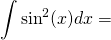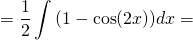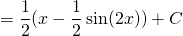# Solution assignment 10 Integration by parts

### Assignment 10

In assignment 3 it was asked to calculate the following integral and we applied integration by parts. Is there another way and if so, which?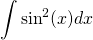### Solution

An important formula from trigonometry is: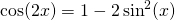Or, written differently: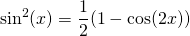This integral can be calculated using standard functions and substitution: